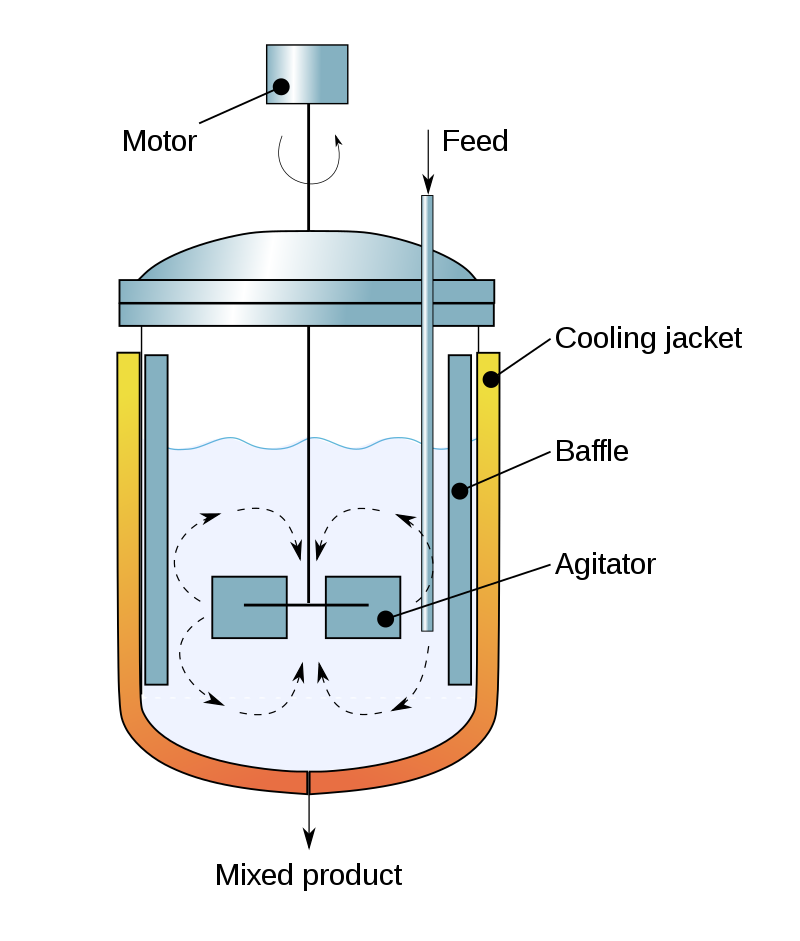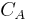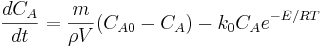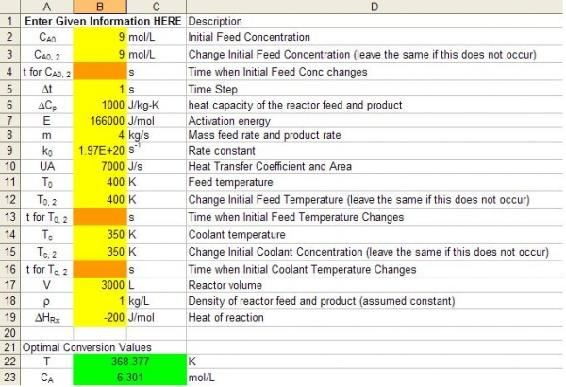# 6.4: ODE and Excel CSTR model with heat exchange

•• Contributed by Peter Woolf et al.
• Assistant Professor (Chemical Engineering) at University of Michigan

Authors: Jason Bourgeois, Michael Kravchenko, Nicholas Parsons, Andrew Wang

## 4.1 Introduction

A Continuous Stirred-Tank Reactor (CSTR) is a chemical reaction vessel in which an impeller continuously stirs the contents ensuring proper mixing of the reagents to achieve a specific output (Figure $$\PageIndex{1}$$). Useful in most all chemical processes, it is a cornerstone to the Chemical Engineering toolkit. Proper knowledge of how to manipulate the equations for control of the CSTR are tantamount to the successful operation and production of desired products. The purpose of this section is to model dynamic conditions within a CSTR for different process conditions. Simplicity within the model is used as the focus is to understand the dynamic control process.Figure $$\PageIndex{1}$$: Cross-sectional diagram of a CSTR. (CC BY-SA 3.0; Daniele Pugliesi via Wikipedia)

## 4.2 Assumptions

For the purposes of this section, we have made the following assumptions to explain CSTR with heat exchange modeling.

• Perfect mixing: The agitator within the CSTR will create an environment of perfect mixing within the vessel. Exit stream will have same concentration and temperature as reactor fluid.
• Single, 1st order reaction: To avoid confusion, complex kinetics are not considered in the following modeling. $\-r_{a}=k C_{A}] • Parameters specified: We assume that the necessary parameters to solve the problem have been specified. • Volume specified: In a control environment, the size of the vessel is usually already specified. • Constant Properties: For this model, we have made the assumption that the properties of the species we are trying to model will remain constant over the temperature range at which we will be looking at. It is important to make this assumption, as otherwise we will be dealing with a much more complex problem that has a varying heat capacity, heat of reaction, etc. ## 4.3 ODE Modeling in Excel To setup the model, the mass and energy balances need to be considered across the reactor. From these energy balances, we will be able to develop relationships for the temperature of the reactor and the concentration of the limiting reactant inside of it. ### 4.3.1 Variable Definitions The following table gives a summary of all of the variables that we used in our mathematical formulas. Symbol Explanations Symbol Meaning Symbol Meaning V Volume of Reactor ρ Density of Stream m Mass Flow Rate CA0 Original Concentration CA Current Concentration T0 Original Temperature T Current Temperature TC Coolant Temperature ΔHrxn Heat of Reaction ΔCp Overall change in Heat Capacity k0 Rate Law Constant E Activation Energy R Ideal Gas Constant UA Overall Heat Transfer Coefficient ### 4.3.2 Mass Balance From our energy and material balances coursework, we know that the general equation for a mass balance in any system is as follows: \[\text {Rate Accumulation}=(\text {Flow } \text {In})-(\text {Flow Out})-( \text {\mathrm{Rate } \text {Generation} ) }$

$\text{Rate Accumulation} =V \frac{d C_{A}}{d t}$

$\text {Flow In}-\text {Flow } \mathrm{Out} =\frac{m}{\rho}\left(C_{A 0}-C_{A}\right)$

$\text{Rate Generation} =V\left(-r_{a}\right)=V C_{A} k_{0} e^{-E / R T}$

In the case of a CSTR, we know that the rate of accumulation will be equal to $$V \frac{d C_{A}}{d t}$$. This comes from the fact that the overall number of moles in the CSTR is $$V C_{A}$$, so the accumulation of moles will just be the differential of this. Sinceis a constant, this can be pulled out of the differential, and we are left with our earlier result. We also know that the flow of moles in versus the flow of moles out is equal to $$\frac{m}{\rho}\left(C_{A 0}-C_{A}\right)$$, which is the mass flow rate, divided by the density of the flow, and then multiplied by the difference in the concentration of moles in the feed stream and the product stream. Finally, we can determine what the rate of generation of moles in the system is by using the Arrhenius Equation. This will give us the rate of generation equal to.

Combining all of these equations and then solving for $$\frac{d C_{A}}{d t}$$, we get that:

$\frac{d C_{A}}{d t}=\frac{m}{\rho V}\left(C_{A 0}-C_{A}\right)-k_{0} C_{A} e^{-E / R T}$

### 4.3.3 Energy Balance

From our thermodynamics coursework, we know that the general equation for an energy balance in any system is as follows:In the case of a CSTR, we know that the rate of energy accumulation within the reactor will be equal to. This equation is basically the total number of moles (mass actually) in the reactor multiplied by the heat capacity and the change in temperature. We also know that the heat generated by this reaction is, which is the rate of mass generation ( − Vra) times the specific heat of reaction (ΔHrxn). The overall rate of heat transfer into and out of the system is given by. This equation is the flow rate multiplied by the heat capacity and the temperature difference, which gives us the total amount of heat flow for the system. Finally, the amount of heat transferred into the system is given by. Combining all of these equations and solving the energy balance for, we get that:In a realistic situation in which many chemical processed deal with multiple reactions and heat effects slight changes to the modeled equation must be done. The diagram below evaluates the heat exchanger under heat effects in which there is an inlet and outlet temperature that is accounted for in the enthalpy term in the newly modeled equation.To model a heat exchanger that accounts for multiple reactions simply take the $$\delta H_{rxn}$$ and $$\delta C_p$$ term and add the Greek letter sigma for summation in front of the terms. When considering a case with multiple reactions and heat effects, the enthalpy and heat capacity of each reaction must be implemented in the energy balance, hence i and j represents the individual reaction species. The equation now looks something like this:### 4.3.4 Euler's Method

In order to model an ODE in Excel, we will be using Euler's Method. The reason why we chose Euler's Method over other methods (such as Runge-Kutta) was due to the simplicity associated with Euler's. Trying to model with methods such as Runge-Kutta are much more difficult in Excel, while Euler's method is quite simple. We also checked the accuracy of our results using Euler's Method by comparing our answers with that of Polymath, a highly accurate ODE modeling program. We found that our answers were the same as that of Polymath, so we are quite comfortable using Euler's Method for our model. Here are the full details on Euler's Method. For this model, we will primarily be interested in the change in concentration of a reactant and the temperature of the reactor. These are the two differential equations that we were able to obtain from the mass and energy balances in the previous section. The following is an application of Euler's method for the CSTR on the change in concentration:

$C_{A i}=C_{A(i-1)}+\frac{d C_{A}}{d t} \Delta t$

This same application can be made to the change in temperature with respect to time:

$T_{i}=T_{i-1}+\frac{d T}{d t} \Delta t$

Assuming that all values in the ODE's remain constant except forand, the new value is then found by taking the pervious value and adding the differential change multiplied by the time step.

### 4.3.5 List of Equations

The following are a summary list of all of the equations to be used when modeling CSTR with a heat exchange.### 4.3.6 Combining Using Excel

To model the CSTR using Excel you use Euler's Method, Energy Balance and Mass Balance together to solve for the concentration at time t.Screenshot of Excel Model for CSTR with Heat Exchange

## 4.4 How to Use Our Model

In order to help facilitate understanding of this process, we have developed an Excel spreadsheet specifically for looking at the changes in concentration and temperature given some change in the input to the CSTR system. An example of a change to the system could be that the temperature of the feed stream has dropped by a given number of degrees, or that the rate at which the feed stream is being delivered has changed by some amount. By using our spreadsheet, you will be able to easily plug in your given parameters, and look at the trend of the concentration and temperature over a wide time interval.

The way in which this spreadsheet works is quite simple. Boxes are provided for you to input all of the given information for your CSTR problem. Various columns containing values for the temperature, concentration, etc., with respect to time have also been provided. There are then more columns that contain the values for the various differential equations from above. With the time derivative in hand, we are then able to predict the value of the temperature or concentration at the next given time interval.

Our easy-to-use Excel model is given here: CSTR Modeling Template. In our model, you will find a column of unknowns that must be specified in order to solve for the optimal conversion temperature and optimal concentration of A. There are then two cells that will display the optimal temperature and concentration. Graphs are also provided to look at the change in temperature and concentration over time. Most of the variables in the model are self-explanatory. One important feature of our model is the option to have a change in the temperature of the feed stream or the concentration of A after a given time t. You do not need to input a value for these cells if there is no change in the feed; it just provides a convenient way to look at the change of temperature and concentration of A. You are also provided with a cell for the time step,. Depending on what size time step you choose, you may need to choose a larger value if your graphs do not reach steady state. If this is the case, the output cells will tell you to increase the time step.

## 4.5 Applications to Process Control

The model created can account for many different situations. However, in process control, it is very important for the following to be considered.

1. Exothermic reaction - In industry, the exothermic reaction is typically of more importance to controls as there is a safety factor involved. (i.e. explosive reactor conditions)
2. Volume constant - For CSTRs, liquids are mostly used.

The model developed can assist in controlling an actual process in many ways including the following, but not limited to:

• Maintaining product concentration despite changes operating conditions
• Predicting and preventing explosive reactor conditions
• Flow rate optimization
• Input concentration optimization
• Operating temperature optimization
• Coolant temperature optimization

This model is very useful for many simple reactor situations and will aid in the understanding of a process and how dynamic situations will affect operation.

In real-life applications, the reaction may not be first order or irreversible or involve multiple reactions. In all these cases, we modify the Energy and Mass Balance with additional rate law considerations.

## 4.6 Worked out Example 1

You are contracted by WOW Chemical to control the operation of their 3000 L jacketed CSTR. They desire to create chemical B from chemical A at an optimal conversion. What is the temperature at which the optimal conversion is achieved?

 A → B is a first order, irreversible reaction. Some information about the process: Feed stream temperature = 400 K Coolant temperature = 350 K Heat of reaction = -200 J/mol Inlet concentration of A = 9 mol/L Inlet flow rate = 4 kg/s Density of A = 1000 g/L UA of the heat exchanger = 7 kcal/s Rate constant = 1.97x1020 s-1 Activation energy = 166 kJ/mol Overall change in Heat capacity = 1 kcal/kg-K.### 4.6.1 Answer to Example Problem #1

From using our handy-dandy worksheet, we see that the optimal conversion occurs at 368.4 K. This comes from inputting all of the given information from the problem statement into the Excel sheet, and then reading off the value for the optimal temperature. Also, make sure you are converting to proper units, such as from kJ to J, for use of our model (just to see if you were paying attention to units).

Example 1 Solution## 4.7 Worked out Example 2

1. In the previous example, the plant was operating in the daytime. At night, there is a drop of 5 K in feed stream temperature. In order to maintain the same concentration do we need to...

A. Decrease the temperature of the cooling water in the heat exchanger.

B. Increase the temperature of the cooling water in the heat exchanger.

C. Maintain the same temperature of the cooling water in the heat exchanger.

2. At night, the inlet flow rate of the system also decreases due to the plant not operating at full capacity. What happens to the temperature of the reaction if concentration of A in the feed stream stays the same?

A. Decrease

B. Increase

C. Stay the same

3. Finally, if the rate constant doubled, what effect will this have on the overall concentration if temperature is held constant?

A. Increase

B. Decrease

C. Stay the same

### 4.7.1 Answers to Example Problem #2

1: B. Since the temperature of the feed stream has decreased, we will need to increase the temperature of the cooling stream in order to maintain the same temperature at which the CSTR was operating at before. Using the Excel spreadsheet from Example 1 and lowering the feed stream temperature by 5 K, we can first see that the temperature of the CSTR has fallen to 366.5 K.We can then adjust the temperature of the feed stream and see that it must be raised by 3 K in order to return to the original operating temperature of 368.4 K.2: A. Since there is less feed coming into the CSTR, the optimal temperature of the reactor will decrease as well. This can again be seen by using our Excel model from Example 1. We know that originally the temperature of the reactor was at 368.4 K. By decreasing the flow rate to 3 kg/s, we can see that the temperature of the reactor decreases by over 3 K down to 365.1 K.3: B. We see in the equations for the differential equations that the rate constant has a negative effect on the conctration of A. By doubling its value, we will then be decreasing CA as well. This can also be seen in the Excel model from Example 1. We see that the original concentration of A is 6.3 mol/L. We can then double the value of the rate constant, and we see that the concentration of A drops down to 4.8 mol/L, which is what we had predicted would happen.## 4.8 References

• Bequette, B. Wayne. Process Dynamics Modeling, Analysis, and Simulation, New Jersey: Prentice Hall.
• Fogler, H. Scott. The Elements of Chemical Reaction Engineering, Prentice Hall. ISBN 0-13-047394-4
• Kravaris, Costas. Chemical Process Control: A Time-Domain Approach, The University of Michigan.
• Riggs, James B.; Karim, M. Nazmul. Chemical and Bio-Process Control, Texas Tech University, Ferret Publishing.